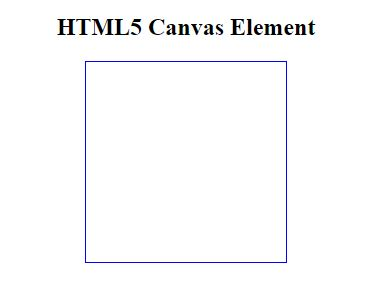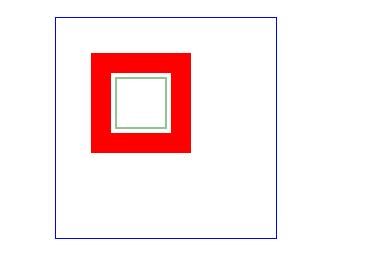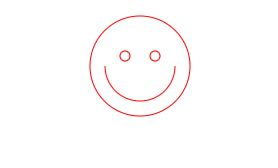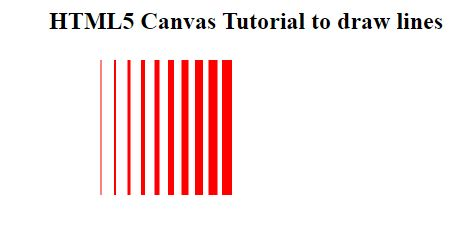# HTML5 Canvas Tutorial - How do i work with HTML Canvas

Published October 25, 2021

Canvas element in the html5 allows us to render dynamic 2d shapes inside webpage using Javascript. In this Html canvas tutorial we will cover create different shapes with html canvas tag. The html <canvas> element tag is used to draw graphics.

We will cover

• HTML Canvas draw line
• HTML canvas rectangle
• HTML canvas draw paths

Let's create different canvas tutorial examples

Draw a HTML Canvas

 ``` Html5 Canvas Tutorial

HTML5 Canvas Element

```

This will give below outputHTML5 Drawing Rectangles

To draw rectangle on the canvas has provided methods

fillRect(x,y,width,height): This canvas method will used to draw a rectangle with fill shape

strokeRect(x,y,width,height): This method will used to create outline rectangle

clearRect() : This canvas method will used to create transparent rectangle

In the above canvas methods x and y params are the position of the canvas from top-left position and width and height are the Canvas height and width respectively.

Canvas Example to Draw simple Rectangle

 ``` ```

Output:Canvas Draw Paths:

 fill()  :  This canvas method will used to Fills the current drawing (path) stroke()  :  This canvas method will used to draws the path you have defined beginPath()    This canvas method will used to Begins a path, or resets the current path moveTo()    This canvas method will used to Moves the path to the specified point in the canvas, without creating a line closePath()    This canvas method will used to Creates a path from the current point back to the starting point lineTo()    This canvas method will used to Adds a new point and creates a line to that point from the last specified point in the canvas clip()    This canvas method will used to Clips a region of any shape and size from the original canvas arc()    This canvas method will used to Creates an arc/curve (used to create circles, or parts of circles) arcTo()    This canvas method will used to Creates an arc/curve between two tangents isPointInPath()    This canvas method will Returns true if the specified point is in the current path, otherwise false

Canvas path example to draw a shape

 ``` ```

output:Canvas Draw Lines

 lineCap()    This canvas method used to Sets or returns the style of the end caps for a line lineJoin()    This canvas method used to Sets or returns the type of corner created, when two lines meet lineWidth()    This canvas method used to Sets or returns the current line width miterLimit() This canvas method used to Sets or returns the maximum miter length

Example to draw lines on canvas

 ```

HTML5 Canvas Tutorial to draw lines

```

Output:Conclusion: In this HTML canvas tutorial we covered how to use html canvas tag to draw rectangle, lines and paths on canvas.

 Article Contributed By :27 Views